# Triangle - math word problems

#### Number of problems found: 1046

• AnnulusTwo concentric circles with radii 1 and 9 surround the annular circle. This ring is inscribed with n circles that do not overlap. Determine the highest possible value of n.
• Regular hexagonal pyramid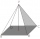Calculate the height of a regular hexagonal pyramid with a base edge of 5 cm and a wall height of w = 20cm. Sketch a picture.Calculate the volume (V) and the surface (S) of a regular quadrilateral prism whose height is 28.6 cm and the deviation of the body diagonal from the base plane is 50°.
• Similarity of two trianglesThe KLM triangle has a side length of k = 6.3cm, l = 8.1cm, m = 11.1cm. The triangle XYZ has a side length of x = 8.4cm, y = 10.8cm, z = 14.8cm. Are triangle KLM and XYZ similar? (write 0 if not, if yes, find and write the coefficient of a similarity)
• Storm and roof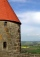The roof on the building is a cone with a height of 3 meters and a radius equal to half the height of the roof. How many m2 of roof need to be repaired if 20% were damaged in a storm?
• Triangle in a squareIn a square ABCD with side a = 6 cm, point E is the center of side AB and point F is the center of side BC. Calculate the size of all angles of the triangle DEF and the lengths of its sides.
• The conicalThe conical candle has a base diameter of 20 cm and a side of 30 cm. How much dm ^ 3 of wax was needed to make it?
• Integer sidesA right triangle with an integer length of two sides has one leg √11 long. How much is its longest side?
• Squares above sidesTwo squares are constructed on two sides of the ABC triangle. The square area above the BC side is 25 cm2. The height vc to the side AB is 3 cm long. The heel P of height vc divides the AB side in a 2: 1 ratio. The AC side is longer than the BC side. Calc
• Land boundaryThe land has the shape of a right triangle. The hypotenuse has a length of 30m. The circumference of the land is 72 meters. What is the length of the remaining sides of the land boundary?
• Two parallel chords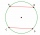The two parallel chords of the circle have the same length of 6 cm and are 8 cm apart. Calculate the radius of the circle.
• Castle towerThe castle tower has a cone-shaped roof with a diameter of 10 meters and a height of 8 meters. Calculate how much m² of coverage is needed to cover it if we must add one-third for the overlap.
• Interior anglesCalculate the interior angles of a triangle that are in the ratio 2: 3: 4.
• Altitude difference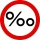What a climb in per mille of the hill long 4 km and the altitude difference is 6 meters?Adam placed the ladder of the house, the upper end reaching to the window at the height of 3.6m, and the lower end standing on level ground and was distant from a wall of 1.5m. What is the length of the ladder?
• Similar trianglesTriangle A'B'C 'is similar to triangle ABC, whose sides are 5 cm, 8 cm, and 7 cm long. What is the length of the sides of the triangle A'B'C ' if its circumference is 80 cm?
• The second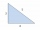The second angle of a triangle is the same size as the first angle. The third angle is 12 degrees larger than the first angle. How large are the angles?
• Cone roofHow many m2 of roofing is needed to cover a cone-shaped roof with a diameter of 10 m and a height of 4 m? Add an extra 4% to the overlays.
• Diamond area from diagonalsIn the diamond ABCD is AB = 4 dm and the length of the diagonal is 6.4 dm long. What is the area of the diamond?
• A kite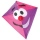Children have a kite on an 80m long rope, which floats above a place 25m from the place where children stand. How high is the dragon floating above the terrain?

Do you have an interesting mathematical word problem that you can't solve it? Submit a math problem, and we can try to solve it.

We will send a solution to your e-mail address. Solved examples are also published here. Please enter the e-mail correctly and check whether you don't have a full mailbox.

Please do not submit problems from current active competitions such as Mathematical Olympiad, correspondence seminars etc...